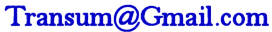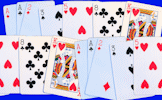# Changing The Subject - Odd One Out

## Which of the five versions of the formula is the odd one out because it is not equivalent to the other four?

##### Level 1Level 2Level 3Level 4Level 5Level 6DescriptionHelpMore Algebra

This is level 3; Formulas which can be rearranged by adding, subtracting, multiplying or dividing both sides by a value. You can earn a trophy if you answer the questions correctly.

 A$$a=b+cd$$ B$$b=a+cd$$ C$$b=a-cd$$ D$$c = \frac{a-b}{d}$$ E$$d = \frac{a-b}{c}$$A$$m=n+3p$$ B$$n=m-3p$$ C$$p = \frac {m-n}{3}$$ D$$\frac{m-n}{p}=3$$ E$$n=m+3p$$A$$t=\frac{u-v}{a}$$ B$$v=u+at$$ C$$u=v-at$$ D$$a=\frac{v-u}{t}$$ E$$t= \frac{v-u}{a}$$A$$t= \frac{g-s}{2}$$ B$$g=t-2s$$ C$$g+2s=t$$ D$$s = \frac{t-g}{2}$$ E$$s = \frac{g-t}{2}$$A$$3x+2y=7$$ B$$3x=7-2y$$ C$$x = \frac{7-2y}{3}$$ D$$y = \frac{7-3x}{2}$$ E$$7+2y=3x$$A$$v=5u-\frac 13$$ B$$3v=5u-1$$ C$$5u-3v=1$$ D$$v=\frac{5u-1}{3}$$ E$$u = \frac{3v+1}{5}$$A$$2a+3b=c$$ B$$a=\frac{c-3b}{2}$$ C$$b = \frac{2a-c}{3}$$ D$$b = \frac{c-2a}{3}$$ E$$c = 2a+3b$$A$$n=\frac{360}{A}-1$$ B$$nA=359$$ C$$nA=360-A$$ D$$nA+A=360$$ E$$n+1=\frac{360}{A}$$This is Changing The Subject - Odd One Out level 3. You can also try:
Level 1 Level 2 Level 4 Level 5 Level 6

## Instructions

Try your best to answer the questions above. Choose one of the five possible answers. When you have finished click the "check" button. If you have any questions wrong, do your best to do corrections but if there is anything you don't understand, please ask your teacher for help.

When you have got all of the questions correct you may want to print out this page and paste it into your exercise book. If you keep your work in an ePortfolio you could take a screen shot of your answers and paste that into your Maths file. You can also claim a 'Transum Trophy' by completing this quiz.

## Transum.org

This web site contains hundreds of free mathematical activities for teachers and students. Click here to go to the main page which links to all of the resources available.

Please contact us if you have any suggestions or Questions.## More Activities:

Comment recorded on the 6 May 'Starter of the Day' page by Natalie, London:

"I am thankful for providing such wonderful starters. They are of immence help and the students enjoy them very much. These starters have saved my time and have made my lessons enjoyable."

Comment recorded on the 21 October 'Starter of the Day' page by Mr Trainor And His P7 Class(All Girls), Mercy Primary School, Belfast:

"My Primary 7 class in Mercy Primary school, Belfast, look forward to your mental maths starters every morning. The variety of material is interesting and exciting and always engages the teacher and pupils. Keep them coming please."

#### Playing Card MathsImagine you are on a desert island with nothing but a pack of playing cards. Do you have to stop learning mathematics? Of course not! Here are some great ideas for teachers, parents and tutors.

## Answers

There are answers to this exercise but they are only available to teachers who have subscribed to Transum and are currently signed in on this computer.

A Transum subscription unlocks the answers to most of the student online exercises, quizzes and puzzles. It also provides the teacher with access to quality external links on each of the Transum topic pages so that teachers can easily find the excellent resources we have found and add to the collection themselves.

Class lists, lesson plans and assessment data can also be stored in the Class Admin application and the teacher also has access to the Transum Trophies earned by class members.

## Go Maths

Learning and understanding Mathematics, at every level, requires learner engagement. Mathematics is not a spectator sport. Sometimes traditional teaching fails to actively involve students. One way to address the problem is through the use of interactive activities and this web site provides many of those. Click here for more activities designed for students in upper Secondary/High school.

Do you have any comments? It is always useful to receive feedback and helps make this free resource even more useful for those learning Mathematics anywhere in the world. Click here to enter your comments.For All:

©1997-2019 WWW.TRANSUM.ORG

## Description of Levels

CloseLevel 1 - Formulas which can be rearranged by adding or subtracting terms from both sides

Example: Make e the subject of the formula d = e - f

Level 2 - Formulas which can be rearranged by multiplying or dividing both sides by a value

Example: Rearrange the formula n = mp

Level 3 - Formulas which can be rearranged by adding, subtracting, multiplying or dividing both sides by a value

Example: Rearrange the formula b = a + cd

Level 4 - Formulas including brackets or expressions in the numerator or denominator of a fraction

Example: Rearrange the formula p = s(t + 2)

Level 5 - Formulas including squares or square roots

Example: Rearrange the formula d² = 2a + 1

Level 6 - Finding the unknown which is not the subject of a formula

Example: If m = n² + 2p, find p when m=8 and n=10

## Help

In level 6, there are two solutions to the questions which involve finding a square root (which could be positive or negative). This program will accept either of the possible answers.

###### Don't wait until you have finished the exercise before you click on the 'Check' button. Click it often as you work through the questions to see if you are answering them correctly.

Close